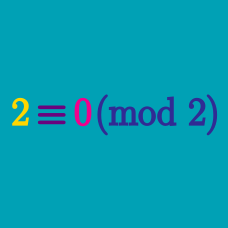Number Theory

# Modular Arithmetic - Multiplication

Given that $2^{20} \equiv 1 \pmod{3} \quad \text{and} \quad 5^{10} \equiv 1 \pmod{3},$ what is the remainder when $$2^{20} \times 5^{10}$$ is divided by 3?

What is $$78 \times 49 \pmod{26}$$?

What is $$\underbrace{\left(6\cdot 6 \cdot 6 \cdots 6\right)}_{\text{15 6's}} \ \bmod{7}?$$

What is equivalent to

$1!+2!+3!+ \cdots + 100! \pmod{12}?$

Give your answer as an integer between 0 and 11, inclusive.

What is $$(10 \times 85) \pmod{5} ?$$

×